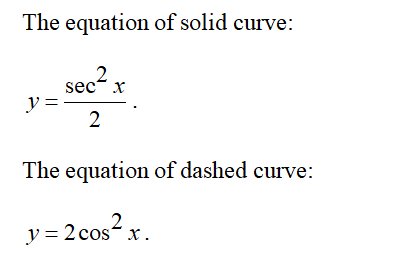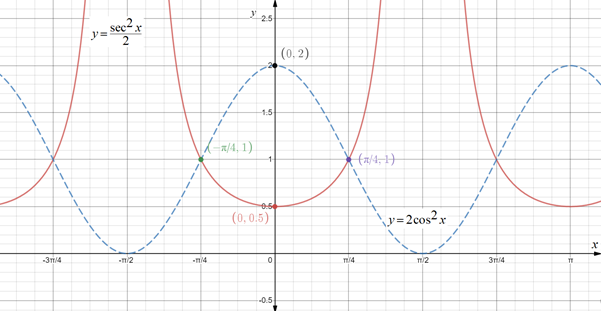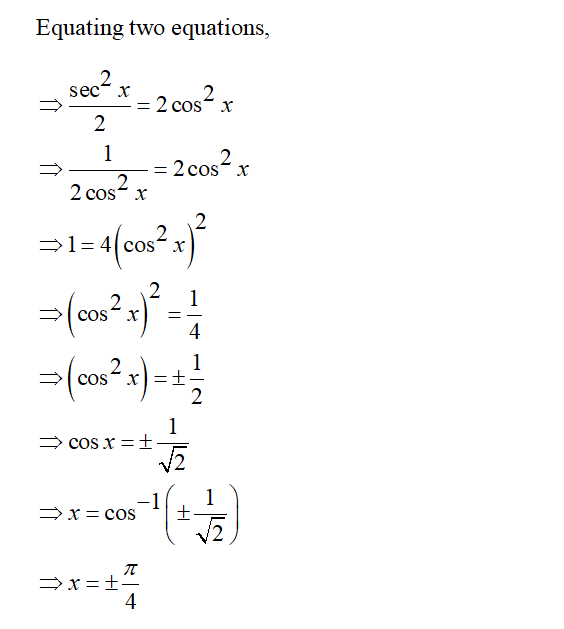# In the figure to the​ right, the equation of the solid curve isy equals StartFraction secant squared x Over 2 EndFractiony=sec2x2and the equation of the dashed curve isy equals 2 cosine squared xy=2cos2x.Determine the area of the shaded region.

Question
51 views

In the figure to the​ right, the equation of the solid curve is

y equals StartFraction secant squared x Over 2 EndFractiony=sec2x2

and the equation of the dashed curve is

y equals 2 cosine squared xy=2cos2x.

Determine the area of the shaded region.

check_circle

Step 1Step 2Step 3...

### Want to see the full answer?

See Solution

#### Want to see this answer and more?

Solutions are written by subject experts who are available 24/7. Questions are typically answered within 1 hour.*

See Solution
*Response times may vary by subject and question.
Tagged in

### Calculus